# Constants in C++

## Constant in C++ with Examples:

In this article, I am going to discuss Constant in C++ with Examples. Please read our previous article where we discussed Template Functions and Classes in C++ with Examples.

##### Constants in C++

As the name suggests, Constants in C++ are given to such variables or values which cannot be modified or changed once they are defined. That means they are fixed values in a program, unlike the variables whose value can be altered. In C++, a constant should be initialized at the time of creating it, and new values cannot be assigned to it later. There can be any types of constants we create in C++ like integer, float, octal, pointers, etc.

There are two ways to define constants in C++. These are as follows:

1. By using the const keyword
2. By using #define preprocessor

In this article, I am going to discuss how to use the const keyword to create constants in C++, and in the next article, I am going to discuss how to use #define preprocessor directives to define constants.

The const is a keyword in C++ which is used at various places to create constants. In this article, I will show you all the places where const can be used to create constants.

##### Using const Inside the main function in C++:

Please have a look at the below code.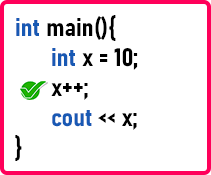Here we have the main function. And inside the main function, first we declared a variable x i.e. int x = 10;. The following is the memory architecture for the variable x.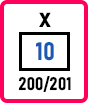Now. can we write x++ here? Yes. So, x will become 11 and will be printed on the screen. This is because x is a normal variable. The complete code is given below.

```#include <iostream>
using namespace std;
int main()
{
int x = 10;
x++;
cout << x;
return 0;
}
```

Output: 11

Now if we write const int x = 10 as follows: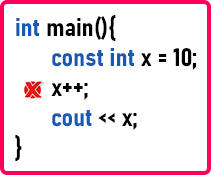Now, x is a constant identifier. x is not a simple variable. A variable is also an identifier, but here x is a constant identifier. So, a constant value means we can’t change it. So, can we write x++ here? No, x is of the const int type, so we cannot change the value of x. If we try to change the value, then we get an error. For a better understanding, please have a look at the below example.

```#include <iostream>
using namespace std;
int main()
{
const int x = 10;
x++;
cout << x;
return 0;
}
```
###### Output: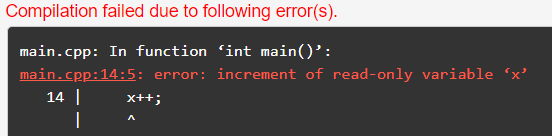So, constant modifiers cannot be modified throughout the program. These variables are also called read-only variables. So, if you have some value and if you don’t want to change it or modify it, then you can declare that value as a constant using the const keyword in C++. It is just like #define,

#define x 10

##### What is the difference between #define and const in C++?

The following are the differences between #define and const in C++.

1. #define is a preprocessor directive and it is performed before the compilation process starts. But const is an identifier that will consume memory for an integer, float, or any type and that memory will have a value that cannot be modified.
2. #define is just a symbolic constant and const is a constant identifier.
3. #define will not consume memory, but const will consume memory according to the data type.
4. #define is not a part of the language, it is pre-compiler whereas const is a part of the language that is part of the compiler.

Note: If you have any constants that are globally used in the project, you can use #define, and if you have any constants inside a function or a class, you can use const qualifier in C++.

Let us look at the next usage of const which is constant pointers.

##### Constant Pointers in C++:

Now we will look at the second usage of constants which is Constant Pointers in C++. Please have a look at the following example.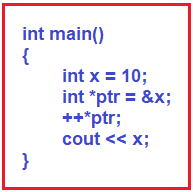Here, we have a variable called x with a value of 10 and a pointer ptr which is pointing at x. Following is the pictorial representation of these two statements;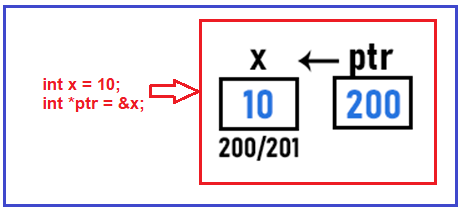Then we have written ++*ptr. So, *ptr will increment the value of x from 10 to 11 as follows.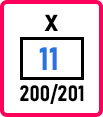Then 11 will be printed on the screen. The following is the complete code example.

```#include <iostream>
using namespace std;
int main()
{
int x = 10;
int *ptr = &x;
++*ptr;
cout << x;
}
```

Output: 11

Now let us understand the same concept using the pointer to a constant. Let us set ptr to const as shown below.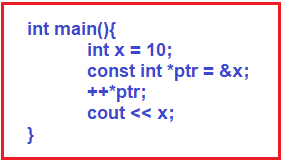So, in this case, the pointer ptr can point to x and access or read x, but it cannot modify the value x. So here, ++*ptr is not allowed. So, by using the constant pointer, we cannot modify the data. But can we say cout << *ptr? Yes. The value 10 will be displayed. So, this pointer can print this value or access x’s value but can not modify the value of x. If we try to modify the value, then we will get an error. The complete example code is given below.

```#include <iostream>
using namespace std;
int main()
{
int x = 10;
const int *ptr = &x;
++*ptr;
cout << x;
}
```
###### Output: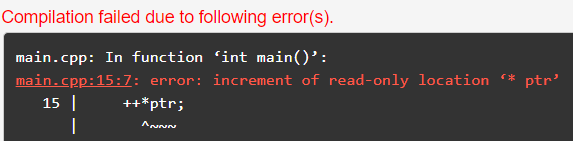Here we have shown you the pointer to an integer constant. This can be written in other ways also, like,

int const *ptr = &x; OR const int *ptr = *x;

Suppose we have one more variable y and it is having a value of 30 (int y = 30;). So, can we point ptr at y? Yes. By writing, ptr = &y, ptr will point at y. So, we can make the pointer point to some other data, but you cannot modify that. Here also, we cannot modify the value of y. For a better understanding, please have a look at the following example.

```#include <iostream>
using namespace std;
int main()
{
int x = 10;
int y = 30;
const int *ptr = &x;
ptr = &y; //Allowed
//++*ptr; //Not Allowed
cout << *ptr;
}
```
##### Another Usage of Constants in C++:

Now let us see the next usage of constants. Instead of writing * after const, let us write it before the const keyword as follows:

int * const ptr = &x;

Now ptr is a constant pointer to an integer. So, who is the constant here? Data is not constant, or an integer is not constant. Pointer ptr is constant here. What does it mean? It means once the pointer ptr is pointing at x, it cannot be changed. After that, you will be unable to change it to another variable. We cannot write prt = &y because ptr is constant. So, the address in that pointer cannot be changed. For better understanding, please have a look at the following example.

```#include <iostream>
using namespace std;
int main()
{
int x = 10;
int y = 30;
int * const ptr = &x;
++*ptr; // Allowed
ptr = &y; //Not Allowed
cout << *ptr;
}
```
###### Output: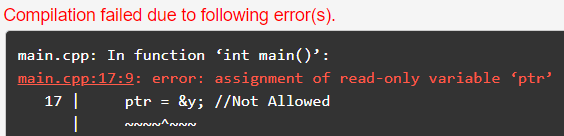So, this is a constant pointer of type integer. A pointer is itself a constant. In the previous example, data was constant, but now the pointer is constant. The pointer cannot be changed to point to any other address in this case.

##### Two constants in C++:

Now let us see the next usage of const. Now, if we write two const like

const int * const ptr = &x;

So, this is a constant pointer to an integer constant. Now, this pointer can not be modified to any other data member, and even though it can not modify the data, i.e., x. So here we can not write the following statements.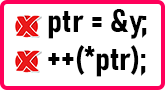We cannot modify the data by writing ++ *ptr and we cannot assign the pointer to another date like ptr = &y. So here we can’t do any of these things. For a better understanding, please have a look at the following example.

```#include <iostream>
using namespace std;
int main()
{
int x = 10;
int y = 30;
const int * const ptr = &x;
++*ptr; //const int * const ptr = &x; Allowed
ptr = &y; //Not Allowed
cout << *ptr;
}
```
###### Output: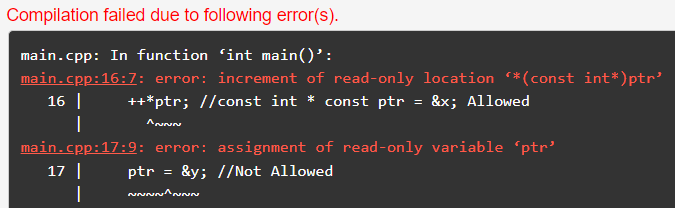##### Types of Constant Pointers in C++

As discussed, there are 3 types of constant pointers in C++. They are as follows.

1. The pointer can be constant: Pointer cannot be modified i.e. ptr = &y is not valid here.
2. Data can be constant: Pointer cannot modify the data i.e. ++*ptr is not valid.
3. Both the pointer and data can be constant: Here pointer cannot be modified i.e. i.e. ptr = &y is not valid as well as a pointer cannot modify the data i.e. ++*ptr is not valid. In this type, we have to write the const keyword 2 times.
##### Const Keyword in C++ Functions:

Now let us look at the next usage of constant keyword i.e. using const in C++ functions. Please have a look at the following code. Here we have a class Demo that has two data members x and y with values of 10 and 20 respectively. Then we have the Display function that will increment the value of x and then print the value of x and y on the screen. Then inside the main function, we have created an object d of class Demo, and we have called the Display function through the object d.

```#include <iostream>
using namespace std;
class Demo
{
public:
int x = 10;
int y = 20;
void Display()
{
x++;
cout << x << " " << y << endl;
}
};

int main()
{
Demo d;
d.Display();
}
```

So, what will be displayed on the screen? At first, x will be incremented to 11 and y will remain the same, so 11 and 20 will be displayed on the screen.

The Display function accesses the data members as well as updates or modifies the data members. If you don’t want the member functions of a class to modify the data members, just read the values and don’t modify the values, then you can write the const keyword after the function name inside the prototype of the function as,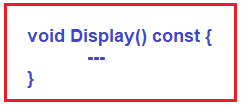Now with the above const keyword in place with the function signature, the compiler will restrict this function from modifying the data member of a class. So, this is the 5th usage of the const keyword. For a better understanding, please have a look at the following example.

```#include <iostream>
using namespace std;
class Demo
{
public:
int x = 10;
int y = 20;
void Display() const
{
x++; //Not Allowed
cout << x << " " << y << endl;
}
};

int main()
{
Demo d;
d.Display();
}
```
###### Output: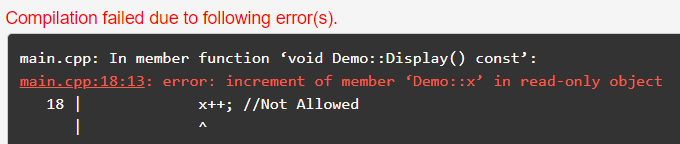Now let us see the next usage of the const keyword. Let us look at the following example.

```#include <iostream>
using namespace std;
void fun(int &x, int &y)
{
x++;
cout << x << " " << y;
}

int main()
{
int a = 10, b = 20;
fun(a, b);
}
```

Here we have a main function in which we have two variables that are a and b with values of 10 and 20. In the next line, we have called the function fun with parameters a and b. In the function fun, we have used the call by reference method. So, x and y will directly access a and b. We already know about the call by reference method that x and y are nothing but aliases of a and b. So, function fun can directly access members a and b.

We’ve also discussed how one function cannot access the variables of another function unless it becomes an inline function. So, the fun code will be copied into the main function which we have called fun. This happens whenever we use “call by reference”. What is the benefit of calling by reference? First, the code is simple for a programmer. Second, that function is a very small function or performs a very simple task, so it can be made inline. And last, the formal parameters will not consume any memory.

But in the call by reference, the function can modify the parameters, which is actually modifying the actual parameters. In the preceding example, we want function fun to be an inline function that does not modify variables a and b. Simply, we’ll put const before the parameter type.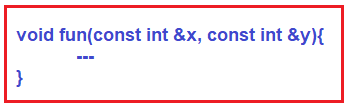So, parameters can also be made constant. Now a and b cannot be changed through function fun. We cannot write x++ or y++ here. For a better understanding, please have a look at the following code.

```#include <iostream>
using namespace std;
void fun(const int &x, const int &y)
{
x++; //Now Allowed
cout << x << " " << y;
}

int main()
{
int a = 10, b = 20;
fun(a, b);
}
```

Output: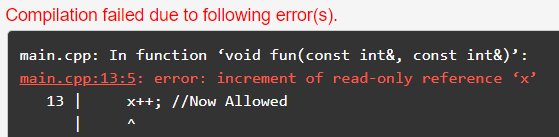So that’s all. These are the usages of the const keyword in C++ Language.